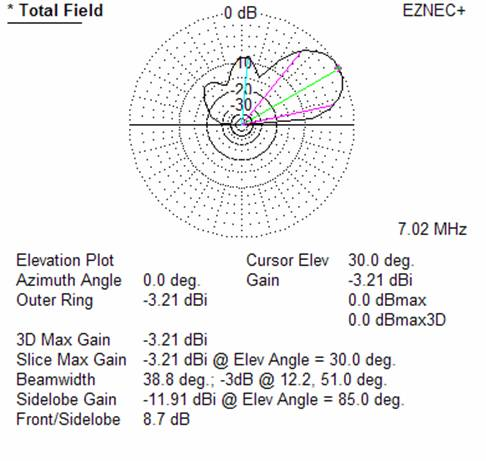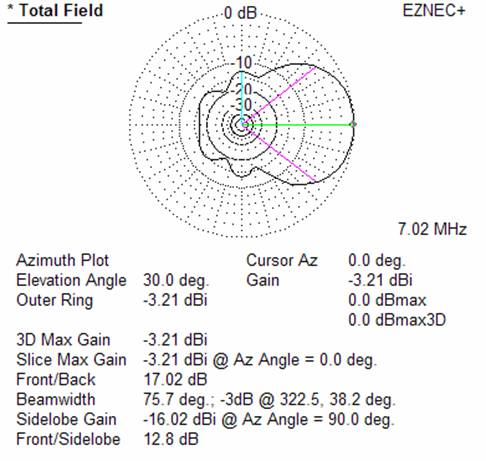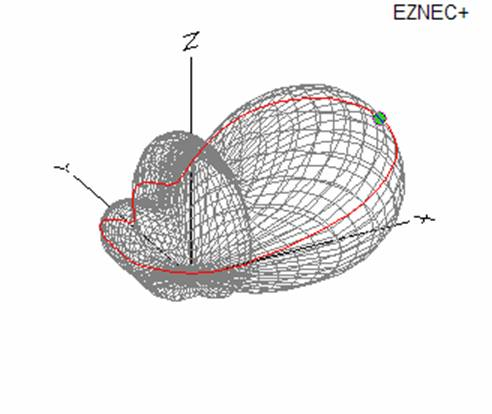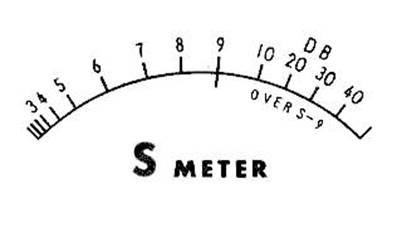Practical Gain

By VE1ZAC

No subject garners more confusion to hams, new and old, than gain, and what it really means. (Thus “Practical” gain)

Gain is often used in the same sentence as transmitter or linear amplifier output, receivers, S meters, or antennas. And, it is almost always described in terms of dB. If you are a ham, you should, by now, have absorbed what the heck a dB is all about. If not, here is a simple way to absorb what it is about. (My apologies to the rigorous among you… this is not!)

Practical dB’s

A db is nothing other than a ratio. It has no dimensions, and isn’t a quantity of anything. In fact, the easiest and really only thing you need to remember about dB is that three of the little doobers  ( 3 dB) is the same as 2 X. ( 2 times, or twice as big). Also, since we are dealing with a number that has to do with powers of a number, -3 of the things (-3dB) means ½.  So, if we talk about something having a gain of 3 dB, we mean it has twice the “Something” of one that doesn’t have a gain of 3 dB.  We can attribute the 3 dB with something else, like direction. Especially when we talk about antennas further on.

Fine, you say, that one is easy. So how do I deal with other dB numbers, for example, 6 dB ? Well.. ask yourself how many 3 dB’s there are in 6 dB ? There are two of them. Each 3 dB is 2 X, so we now have 2x 2x which is 4 times. Ahhhh… 6 dB means something is 4 times as big.  This works because we are talking about arithmetic on powers of a base number. To multiply, we add the powers.

What about 10 dB ?  There are 3 of the 3 dB’s in there at 2 X each, for a total of 8 x and  1 dB left over. You know the “Times” in this case has to be less than 2 and greater than 1.. so if you guess at something in between you will be pretty close. Let’s use 1.25.  In fact 10 dB is conveniently 10 x. Another good one to remember.

Minus dB’s are simply dividing instead of multiplying. This -6 dB means ¼ and -10 dB means 1/10.

Practical transmitter power

We often talk about amplifiers as having gain. For example, my shiny new linear amplifier might have a gain of 10 dB.  That means that if we drive it with our basic 100 watt transceiver output, it should produce a power of 100 x 10 = 1000 watts, or 1 kW. (Assuming, of course, that it is OK to drive it with a signal this large)

Practical antenna gain

I have an antenna that has a gain of 6 dB.  What does that mean? Well, first off, we need to know where that gain is, since it is an antenna. It might be in one direction, it might be everywhere. We need to ask where it is. If it is a beam, it likely has gain in it’s forward direction. Then, 6 dB means any received signal will be 4 times as big, and anything transmitted in that same direction will appear that it has an ERP, or effective radiated power 4 times as large.  We could get the same result, at an observer’s receiver, by using a ‘no gain’ antenna and using a 6 dB amplifier at our transmitter. The observer wouldn’t know the difference.  (Keep in mind that these beam type directions are often VERY specific…. That is, a certain direction, and certain elevation above the horizon… thus the need to be able to point our beam in various directions)Another number that comes up with beams is the F/B (front to back) ratio. This is the difference in forward and reverse gain from the same beam. So, our same beam we used above might have a F/B ratio of 15 dB (not unusual, by the way). That means we have 2x 2x 2x 2x 2x gain = 32 times gain one way compared to the other. Or, if we look in the backward direction, we reduce reception and transmission to 1/32.  Pretty small. Great for eliminating a source of interference, by pointing away from it.Here are some EZNEC model outputs of a short Beverage antenna recently undertaken at the authors QTH.  (This Beverage is a 200 feet long wire 6 feet high, and terminated with a resistor to ground. Slightly different from the one built) Notice all the dB gain information and how much effort is taken to demonstrate the direction of the gain numbers. The green line is the particular direction and point we are describing. BTW, that point can be moved around all through the model to probe gain in any direction in the field. You can see that the gain changes all over the field. It is obvious that any number we refer to needs some direction information.Another term has come up: dBi.  This means dB gain referenced to an isotropic antenna. Sometimes we use dBd, which refers the gain to a classic dipole. That one means a lot to some people, because they understand dipoles well.

Here is a 3D view of the same antenna. These views represent a macro or far field view of the antenna field. Imagine it laying over the Earth.

What is that S meter telling us ?

There are two receiver issues dealing with gain. The first is the poorly understood and oft quoted S meter. What the heck is an S meter, what is it really telling us anyway?

An S meter is a fairly poorly engineered measurement device which is supposed to tell us, very roughly, what the gain of a received signal is, compared to a shorted antenna input. The scale is arbitrarily divided up into 9 S units (1 to 9) with the far left needle position being 0. Beyond that, there are a few more divisions that are marketed in dB, often inunits of 10 dB. These might go up to +50 or 60 dB.

S unit calibrations are, unfortunately, not completely uniform. However, by and large, receiver manufacturers try and have the value of one S unit = 6 dB. There are some that go as low as 4 dB.  In our discussion we will use 6 dB, but be forewarned that this might not apply to every transceiver o or receiver out there. There is also another loose standard that says “S9 shall be equivalent to a 50 uVolt (micro volt, or 50 X .000001 volt) signal on the antenna terminals with a 50 ohm load”

Remembering our ability to add dB’s to get equivalent gain meanings, S9 means 9x6x, or 54db, or 2 to the power of 18 times more signal than what would be present with a shorted antenna input. All of us know in practical terms that if we have background noise of 2 S units, and the signal we find very  clear is 4 S units, the difference is 2 S units,. This means our received signal is 12 dB louder than the noise. If our signal is S9 plus 20 dB, that would be 54 dB plus 20 dB = 74 dB, which now means 2 to the power of almost 25. A really big number.  (With numbers this awkward, you might now get an inkling of why we want to use dB at all…. It’s because the numbers are much easier for our brains to deal with, than say 2 to the power of 25!)

In practical terms… if we are now at a receiver and watching a signal from our friend with the two antennas, we might see a S meter reading of 4 on his signal with a ‘no gain’ antenna, and when he switches to the 6 dB beam, we see the signal go up by one S unit ( 6 dB) to S5. That’s all it means.

The same situation might occur with an amplifier. Without the amp, the signal is S4. With the 10 dB amp in play, the signal goes up 10 dB, or maybe 1 ½ S units.

Here is another example: QRP (5 watts) vs. QRO ( 100 watts). What does it mean? Well, 5 watts times 20 = 100 watts, 10x is 10 dB and 2x is 3 dB, leaves us with 13 dB difference. What does that mean for the listener and his receiver?  2+ S units… that’s all. Puts things in perspective!

(I hope there is a round of “Ah-hah’s” being heard out there.)

Remember, that these S meters are seldom very accurate, and two different rigs with the same input may or may not show the same S number. For that reason, it is a big mistake to give signal reports with   S units. Use the objective RST reporting system and state what your ears are hearing, not what your eyes are seeing. It will mean a lot more to the other ham.

Practical Receiver gain and dynamic range numbers

Manufacturers often report their receiver gain numbers in glowing terms, expecting the purchaser to equate big numbers with better. This often is misleading.  Remember that when we amplify our antenna signal, we are also amplifying the noise that is attendant with the signal. So cranking the whole thing up isn’t going to be much use unless we can figure out how to pick our signal out of the noise. The ability of the receiver to do that has less to do with gain and more to do with operator controls. (Take a look at VE1RGB’s article on this website.) The gain number must refer to something, and a quality manufacturer will refer to gain of a signal with a certain SNR  or signal to noise ratio. Remember our example of the signal 2 S units above the noise ?

Caution: SNR calculations are different for power dBs and voltage level dBs. Another discussion subject.

Dynamic range is a more useful number, and often tells a practical story about a receiver. Here the dB number will refer to the change in signal strength “Range” that the receiver is capable of dealing with under a set of good conditions. A high number here is a good thing, and often difficult to obtain. Surprisingly, many complicated receivers may have a worse value for dynamic range than a very simple receiver. Something to keep in mind.

There are other receiver and transmitter measurements that use dB as well. That may be the subject of other articles in the future.

For now, let’s recognize the ubiquitous “dB” as a practical hams friend from the math world.